Hu, J., Lei, T., Shen, S., and Zhang, Q. (2013). "Specific energy consumption regression and process parameters optimization in wet-briquetting of rice straw at normal temperature," BioRes. 8(1), 663-675.

#### Abstract

In the normal-temperature (20 to 25 ˚C) wet briquetting of straw, the lignin becomes softened and briquetted by means of friction heat from the briquetting at some compressing force and moisture ratio. With an electronic universal testing machine and a self-developed normal- temperature wet briquetting device, tests were carried out for normal-temperature wet briquetting of rice straws with different compressing rate, material moisture, die length-diameter ratio, and die opening taper, using a quadratic regression-orthogonal design. Based on the characteristic curves of normal-temperature wet briquetting, the specific energy consumption was calculated by stepped regression and then integration for summation. Thereby, a specific energy consumption model was established. Next, an interactive analysis was made between single-factor and two-factor designs. Optimal combination of factors, i.e. 17.3% moisture, 44.5 mm/min compressing rate, 45˚ die opening taper, and 5.3 die length-diameter ratio, were obtained while the specific energy consumption was minimized. Further, the work provided references for process parameters design of the normal-temperature wet briquetting device for straw.

Specific Energy Consumption Regression and Process Parameters Optimization in Wet-Briquetting of Rice Straw at Normal Temperature

Jianjun Hu,a Tingzhou Lei,b Shengqiang Shen,c and Quanguo Zhang a,*

In the normal-temperature (20 to 25 oC) wet briquetting of straw, the lignin becomes softened and briquetted by means of friction heat from the briquetting at some compressing force and moisture ratio. With an electronic universal testing machine and a self-developed normal- temperature wet briquetting device, tests were carried out for normal-temperature wet briquetting of rice straws with different compressing rate, material moisture, die length-diameter ratio, and die opening taper, using a quadratic regression-orthogonal design. Based on the characteristic curves of normal-temperature wet briquetting, the specific energy consumption was calculated by stepped regression and then integration for summation. Thereby, a specific energy consumption model was established. Next, an interactive analysis was made between single-factor and two-factor designs. Optimal combination of factors, i.e. 17.3% moisture, 44.5 mm/min compressing rate, 45o die opening taper, and 5.3 die length-diameter ratio, were obtained while the specific energy consumption was minimized. Further, the work provided references for process parameters design of the normal-temperature wet briquetting device for straw.

Keywords: Rice straw; Wet-briquetting; Specific energy consumption; Die length-diameter ratio; Die opening taper

Contact information: a: Department of Mechanical & Electrical Engineering, Henan Agriculture University, Zhouzhou 450008,Henan,China; b: Henan Academy of Sciences, Zhengzhou 450008,Henan,China; c: School of Energy and Power Engineering, Dalian University of Technology, Dalian 116026,Liaoning China;*Corresponding author: zquanguo@163.com

INTRODUCTION

Utilization of straw resources is becoming an important strategy in the field of energy, and also an important option for fuel replacement and emission reduction. The straw briquetting increases the raw material density to 1×10kg/m3, thus faciliating straw transport and storage. The energy density of straw briquettes is equivalent to that of coal with medium heat value; straw briquettes can replace coal and liquid gas as cooking energy and industrially replace coal used in boilers. Thus straw briquetting is emerging as a key to the utilization of crop straw as an energy resource.

Current domestic and foreign briquetting methods of straws are divided into closed compression and open compression (Chen et al.2009; Felfli et al. 2011). The closed compression is a discontinuous briquetting process which is focused on the investigation of straw briquetting mechanisms and rules; the open compression is a continuous production process that is applied in actual production and is focused on optimization of straw briquetting process parameters. As for open compression, the straw briquetting process is divided into normal-temperature wet briquetting, hot briquetting, and carbonization briquetting (Wu et al. 2010).

In actual production, essentially all straw briquetting equipment provides open compression, so it is especially useful to research the open briquetting of straws. In terms of open briquetting, the specific energy consumption is one of the most important performance indicators to check whether the briquetting equipment is economical. Specific energy consumption refers to the ratio of energy consumption, arising from briquettes production, per unit time, to briquettes mass produced in that time (Li et al. 2011). In China, the normal-temperature wet briquetting process is mostly used in commercial applications. The process is performed without external heat. The energy consumption from straw briquetting consists of two components: 1) energy consumption for overcoming internal friction between the material and the briquetting device; 2) energy consumption for overcoming material deformation (Mani et al. 2006). The improper design of process parameters of normal-temperature wet briquetting equipment on the market causes high specific energy consumption, which limits their application and promotion to a significant extent.

The utilization of straw will be expected to exceed 80% by 2015 in many countries, particularly in China. Rice is widely planted in China. The biomass in rice straw accounts for approximately 62% of crop residues, and it is the greatest biomass resource in China. In this article, a universal testing machine and a self-developed open-type briquetting device were used for normal-temperature wet briquetting experimental research with rice straw with respect to different process parameters, and then the optimal combination of process parameters to minimize the specific energy consumption was analyzed. This provided a reference basis for design of process parameters of normal-temperature wet briquetting equipment.

Experimental

Material

The experimental material in this article is rice straws, as commonly seen in Xinyang, China. The rice straw was crushed by a TF-9FX-50 granulator of 4-mm mesh, manufactured by the Midwest Technology Co., Ltd. Then foreign materials were removed from the crushed straws. Next, the straws were dried in a muffle furnace at 105 ˚C for 8 h and then classified for storage. When the temperature of the straws decreased to room temperature, a water distribution test was performed on request. Thirty grams of the test sample of each type was taken. By calculation of each water addition, the moisture ratios of materials were controlled, and then the materials were sealed and stored in black plastic bags in accordance with different moisture ratios.

Device

The experimental device used in this work was a WDW-50 universal computer- controlled electronic tester produced by the Jinan Start Testing Technology Co., Ltd. The device is hydraulically powered. It can adjust its compressing rate and automatically collect parameters including compressing force and displacement. The measurement of experimental compressing force is within the range of 1 to 50 kN, the movement speed of beam is 2.0 to 200.0 mm/min, and the accuracy of displacement measurement is ±1%. Aiming at characteristics of the normal-temperature wet briquetting, a set of experimental devices was designed, which includes a sleeve, a die, a compression bar, a connection pin, a fixture, a seat, etc., as shown in Fig.1.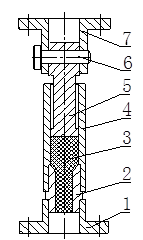1. base 2. die 3. material 4. sleeve 5. compression bar 6. connection pin 7. fixture

Fig. 1. Experimental device in the normal-temperature wet briquetting of rice straws

In this experiment, the inside diameter of the sleeve was designed to be 15 mm; the die was designed as a 10 mm inside-diameter cylinder with its opening of a certain taper. The length-diameter ratio of die was defined as the ratio of its height to its inside diameter at the section of cylinder, and the ratio was determined by adjusting the height while keeping the inside diameter constant.

Experimental Method

Before the experiment, the material was weighed with an electronic balance and put into the sleeve. Then it was pre-pressed using a stamp stem, with the purpose to pro-

Table 1. Factor Level Codes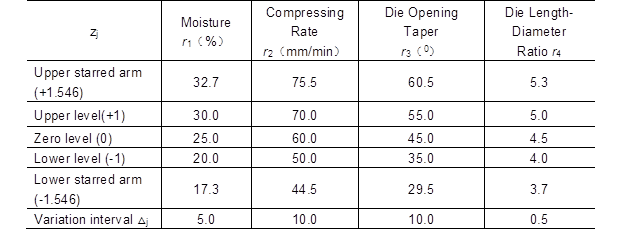In Table1,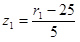,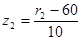,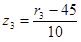,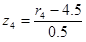.

vide an initial material thickness of 115 mm. The compressing force rating in the experiment was set to 50 kN. By setting to different compressing rates and starting the universal tester process, curves of compressing force (P) and piston displacement (x) could be obtained automatically.

In the normal-temperature wet briquetting of straws, important parameters having influence on specific energy consumption included compressing rate, material moisture ratio, die length-diameter ratio, and die opening taper (Nilsson et al. 2011). Their relationship with the specific energy consumption was not simply linear but showed interaction among them to different extent (Andrejko and Grochowicz 2007; Adapa et al. 2009). Therefore, the quadratic regression orthogonal design was used for testing (Ndiema et al. 2002), with factor level codes shown in Table 1.

RESULTS AND DISCUSSION

Approximate Calculation of Specific Energy Consumption

From the P-x curve in each normal-temperature wet briquetting, different material moisture ratios, compressing rates, die length-diameter ratios, and die opening tapers showed the same changing rule of characteristic curve in the normal-temperature wet briquetting, as shown in Fig. 2.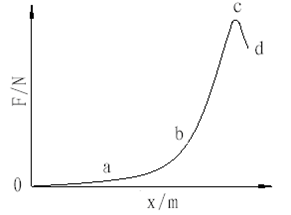Fig. 2. Characteristic curve in the normal-temperature wet briquetting of rice straws

The curve in Fig. 2 shows a complex wet briquetting process for rice straws. To facilitate the data calculation of specific energy consumption, the wet briquetting process was divided into loose stage (oa), transition stage (ab), compression stage (bc), and evolution stage (cd). The four stages showed different briquetting mechanisms: elastic deformation of the straws occurred in the loose stage and the transition stage, plastic deformation of the straws occurred in the compression stage, and stress relaxation of the straws occurred in the evolution stage (Ndiema et al. 2002).

In this article the specific energy consumption was calculated using the curve area formed by compressing force and displacement. The energy consumption was calculated as follows: Regression analysis was performed with experimental data of each wet briquetting in four stages using the software Excel, which yielded the regression equation of each stage and substituted it into Equation (1) for approximate calculation of the energy consumption (Hu 2008). The specific energy consumption during the whole briquetting process was calculated by dividing the above-mentioned energy consumption by the mass of the straws.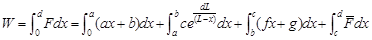(1)

In Equation (1), F is the compressing force of the compression bar (N); X is the displacement of the piston (m); abcdf, and g are experimental coefficients; L is the sleeve height (m); and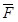is the average compressing force of straw when showing stress relaxation (N).

Experimental Results of Specific Energy Consumption

By using the quadratic regression orthogonal design, research on the normal- temperature wet briquetting experiments of rice straws was performed, and experimental results are shown in Table 2.

In Table 2,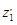=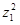-0.77,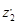=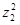-0.77,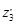=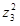-0.77, and the Uj value corresponding to the sign “△” is incorporated into the remainder term.

Establishment of Regression Model of Specific Energy Consumption

By using experimental result yi in Table 2, relevant parameters (e.gBjdjbjUj, and Fj) were calculated with the formulae below (Yuan et al. 2001):

Calculation of Bj: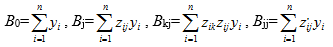(2)

Calculation of bj: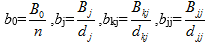(3)

In Equation (3),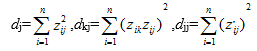(4)

Calculation of Uj: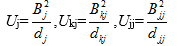(5)

Check of the regression equation for significance:

Total sum of squares：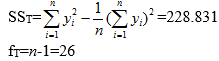(6)

fT=n-1=26

Subsume the smaller term of Uj under the remaining sum of squares Qe2, and in the experiment f= 9.

Table 2. Results of Quadratic Regression Orthogonal Experiments with Four Factors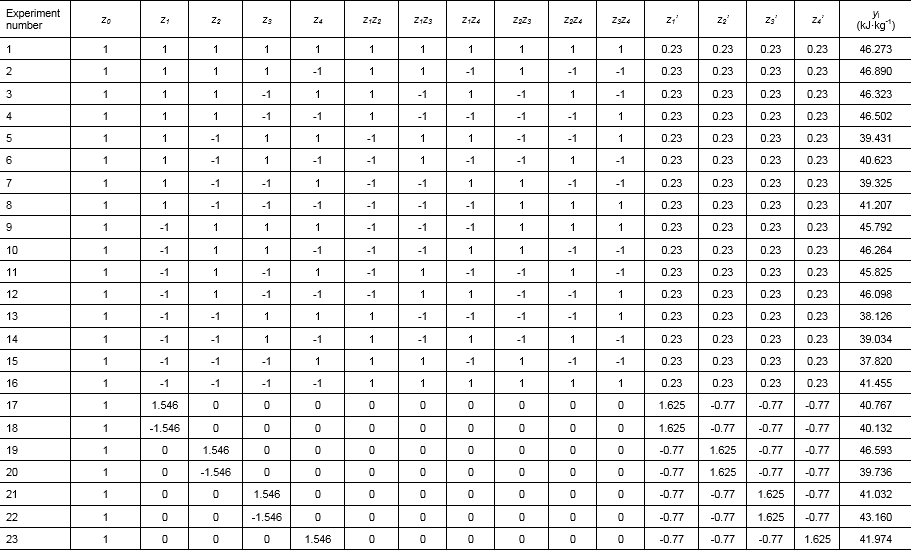Table 2. continued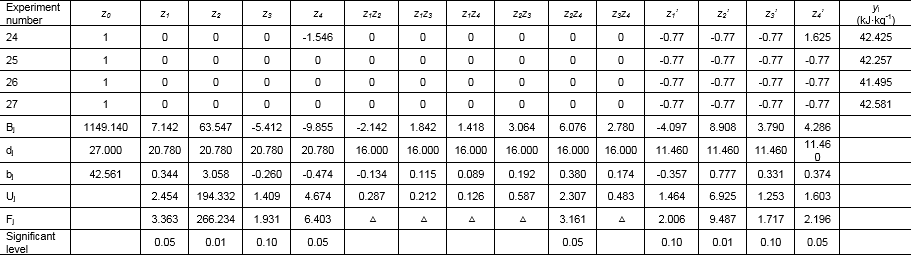Qe2= SSTU=12.409 (7)

In Equation (7),

U=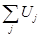=216.422 (8)

fe2fTfU=17 (9)

MSe2Qe2fe2=0.730 (10)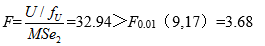(11)

Check of regression coefficients for significance: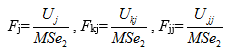(12)

Calculation results are listed in Table 2. The regression coefficients were significant to different extent, as shown.

The check for degree of fitting was as follows:

Sum of error squares was obtained from zero-level experimental results.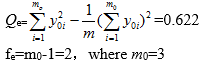(13)

Sum of squares of unfitting terms:

QLf=Qe2Qe=11.787 (14)

fLf=fe2fe=15 (15)

FLf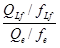=2.53＜F0.05（15,2）=19.43 (16)

Calculation results showed good fitting in the regression equation, which can be expressed as:

y=41.694+0.344z1+3.058z2-0.26z3-0.474z4+0.38z2z4-0.357z12+

0.777z22+0.331z32+0.374z42 (17)

Analysis of Regression Model of Specific Energy Consumption

Analysis of main effect

In the quadratic regression, orthogonal design factors were processed with non- dimensional linear codes, and there was no relation between regression coefficients bj of the first-order term as well as between regression coefficients of interactive terms and the quadratic term. Therefore, the value of absolute regression coefficients was used to compare influence of the first-order term on the specific energy consumption. In the experiment, z2>z4>z1>z3i.e. the compressing rate, had the greatest influence on the specific energy consumption, the die length-diameter ratio had the greater influence, and the die opening taper had the smallest influence. The higher the compressing rate and the moisture ratio, the greater the specific energy consumption; but the die length-diameter ratio and the die opening taper had different tendencies from those of the compressing rate and moisture ratio.

Analysis of single-factor effect

Fixing three of four factors in the regression model to the zero level yielded the single-factor model below:

Material moisture ratio:

y1=41.694+0.344z1-0.357z12 (18)

Compressing rate:

y2=41.694+3.058z2+0.777z22 (19)

Die opening taper:

y3=41.694-0.26z3+0.331z32 (20)

Die length-diameter ratio:

y4=41.694-0.474z4+0.374z42 (21)

In Equations (18) through (21), successive assigning zj=-1.546, -1, 0, 1, and 1.546 yielded respective yi, and regression equation curves characterized by single factors and specific energy consumption were plotted in Fig. 3.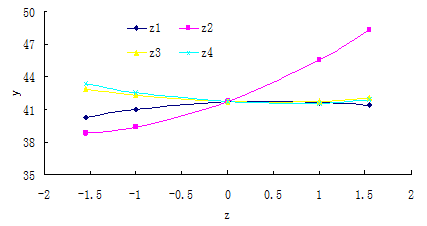Fig. 3. Regression equation curves characterized by single factors and specific energy consumption

Figure 3 shows different trends of regression equation curves characterized by single factors and specific energy consumption. As to z1, when the compressing rate, die opening taper, and die length-diameter ratio are in zero level, the specific energy consumption increases and then decreases with the increasing moisture ratio; as to z2, when the moisture ratio, die opening taper, and die length-diameter ratio are in zero level, the specific energy consumption increases with the increasing compressing rate; as to z1 and z4, when other three factors are in zero level, the specific energy consumption decreases and then increases but by a small amount.

Analysis of Marginal Specific Energy Consumption Effect by Single Factors

Derivation of the above-mentioned four single factors and respective assigning zj=-1.546, -1, 0, 1, and 1.546 yielded the marginal specific energy consumption. The marginal specific energy consumption effect by single factors in different levels is shown in Fig. 4.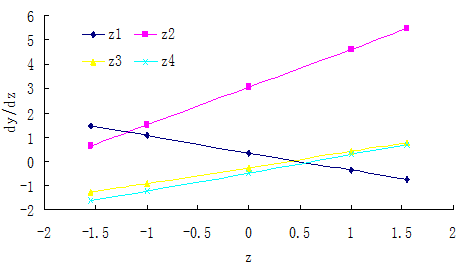Fig. 4. Marginal specific energy consumption effect by single factors

As shown in Fig. 4, the moisture ratio and the compressing rate exhibits greater influence on the marginal specific energy consumption: the greater the moisture ratio, the lower the marginal specific energy consumption; the greater the compressing rate, the higher the marginal specific energy consumption. As far as the die opening taper and the die length-diameter ratio are concerned, they show an approximate trend that is represented by the increasing marginal specific energy consumption (but by a small amount) as the die opening taper and the die length-diameter ratio increase. Figure 4 also shows that the factors have different influence on the specific energy consumption when in different level. This provides references for selection of specific energy consumption reduction factors and determination of process parameters in different conditions (Hu 2008).

Analysis of Interaction between Two Factors

It is learned from Equation (17) that the interaction of z2 and z4 is most significant but the interaction of other two factors is less significant. Only the interaction of z2 and z4 is, therefore, analyzed in this article. Fixing z1 and z3 to the zero level yielded the following equation:

yz2z4=41.694+3.058z2-0.474z4+0.38z2z4+0.777z22+0.374z4(22)

Substituting the codes of z2 and z4 in each level into Equation (22) yielded yz2z4, as shown in Table 3.

Table 3. Interaction of Compressing Rate and Die Length-Diameter Ratio on Specific Energy Consumption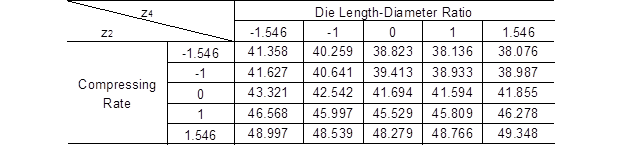It can be seen in Table 3 that no matter which level the die length-diameter ratio is in, the specific energy consumption increases with the increasing compressing rate and the increment also increases; when the compressing rate is in the level of -1.546, the specific energy consumption decreases with the increasing die length-diameter ratio and the decrement also decreases; when the compressing rate is in the level of -1, 0, 1, and 1.546, the specific energy consumption decreases and then increases with the increasing die length-diameter ratio. When the compressing rate is in the level of -1.546 and the die length-diameter ratio is in the level of 1.546. This is to say, if the compressing rate is 44.5 mm/min and the die length-diameter ratio is 5.3, then the specific energy consumption reaches its lowest value of 38.076 kJ/kg.

With the extreme theory of multi-variable functions, yz2z4 in Equation (22) was partially differentiated against z2 and z4, and then the two differentiated yz2z4 were assigned zero, yielding: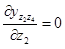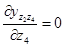(23)

Then z= -2.424 (equivalently the compressing rate was 35.8 mm/min) and z= 1.863 (equivalently the die length-diameter ratio was 5.4), and the specific energy consumption reached its minimal value of 37.546 kJ/kg.

Optimization of Process Parameters

With the statistical optimization method, the optimal combination of factors was determined. Each factor was assigned five levels of ±1.215, ±1, and 0. The software Matlab was used to search 5= 625 schemes for the optimal process scheme with the lowest specific energy consumption, i.ez= -1.546, z= -1.546, z= 0, and z= 1.546, which were equivalently 17.3% in moisture ratio, 44.5 mm/min in compressing rate, 45o in die opening taper, and 5.3 in die length-diameter ratio; in such case, the specific energy consumption was 36.691kJ/kg. This approximated to the conclusion from reference (Hu et al. 2008) that the moisture ratio was 16%, the compressing rate was 45 mm/min, the die opening taper was 45o, and the die length-diameter ratio was 5.2. The difference in moisture ratio is due to wheat straws used in reference (Hu et al.2008), and wheat straws and rice straws have different biological structure, which is the major cause for the difference.

CONCLUSIONS

1. In this article, a quadratic regression orthogonal design is used to perform research on the normal-temperature wet briquetting of rice straws, and results show that the normal-temperature wet briquetting of rice straws has similar changing rule in the conditions of different process parameters. The normal-temperature wet briquetting is divided into a loose stage, a transition stage, a compressing stage, and an evolution stage, and the specific energy consumption is calculated with the method of staged regression followed by integral for summation.

2. Results of the analysis of main effect in the specific energy consumption regression model show that the compressing rate, material moisture ratio, die length-diameter ratio, and the die opening taper have different influence on the specific energy consumption. The compressing rate has the greatest influence on the specific energy consumption, while the die opening taper has the smallest influence on the specific energy consumption; additionally, the effect of the compressing rate and moisture is positive, while the effect of the die length-diameter ratio and die opening taper is negative.

3. Results of analysis of single-factor effect in the specific energy consumption regression model show that, with the increasing moisture ratio, the specific energy consumption increases and then decreases; with the increasing compressing rate, the specific energy consumption increases sharply; with the increasing die opening taper or die length-diameter ratio, the specific energy consumption decreases and then increases.

4. Results of analysis of single-factors’ marginal specific energy consumption effect in the specific energy consumption show that the factors have different effects on the marginal specific energy consumption: the moisture ratio and the compressing rate have greater influence on the marginal specific energy consumption, while the die opening taper and the die length-diameter ratio have smaller influence.

5. Results of analysis of interaction between two factors show that the interaction between the compressing rate and the die length-diameter ratio on the specific energy consumption is significant, while the interaction between other factors is insignificant. The specific energy consumption reaches its lowest valve when the compressing rate is in the level of -1.546 and the die length-diameter ratio is in the level of 1.546.

6. Results of the optimization from 625 schemes with the software Matlab show that the optimal combination of factors with the lowest specific energy consumption is a moisture of 17.3%, a compressing rate of 44.5 mm/min, a die opening taper of 45o, and a die length-diameter ratio of 5.3; in such case, the specific energy consumption is 36.691 kJ/kg. This conclusion coincides well with research results in relevant literature.

ACKNOWLEDGMENTS

This work was financially supported by the Chinese National ‘863’ Project (2001AA514010) and the Henan Natural Science Foundation (0611021100).

REFERENCES CITED

Adapa, P., Tabil, L., and Schoenau, G. (2009). “Compaction characteristics of barley, canola, oat and wheat straw,” Biosystems Engineering 104(3), 335-344.

Andrejko, D., and Grochowicz, J. (2007). “Effect of the moisture content on compression energy and strength characteristic of lupine briquettes,” Journal of Food Engineering 83(1), 116-120.

Chen, L. J., Xing, L., and Han, L. J. (2009). “Renewable energy from agro-residues in China: Solid biofuels and biomass briquetting technology,” Renewable and Sustainable Energy Reviews 13(9), 2689-2695.

Felfli, F. F., Mesa, P. J. M., Rocha, J. D., Filippetto, D., Luengo, C. A., and Pippo, W. A. (2011). “Biomass briquetting and its perspectives in Brazil,” Biomass and Bioenergy 35(1), 236-242.

Hu, J. J. (2008). “Experimental study and numeric simulation on straw pellet fuel cold compressing molding,” Ph.D. Thesis. Dalian University of Technology.

Hu, J. J., Lei, T. Z., He, X. F., Li, Z. F., Liu, J. W., Wang, Z. W., Zhu, J. L. (2008). “Experimental research on the wheat straw pellet fuel compressing molding parameter under cold conditions, ” Acta Energiae Solaris Sinica (Tai Yangneng Xuebao) 29(2), 241-245.

Li, F. M., and Zhang, M. Q. (2011). “Technological parameters of biomass briquetting of macrophytes in Nansi Lake,” Energy Procedia 5, 2449-2454.

Mani, S, Tabil, L. G., and Sokhansanj, S. (2006). “Specific energy requirement for compacting corn stover,” Bioresource Technology 97(12), 1420-1426.

Ndiema, C. K. W., Manga, P. N., and Ruttoh, C. R. (2002). “Influence of die pressure on relaxation characteristics of briquetted biomass,” Energy Conversion and Management 43(16), 2157-2161.

Nilsson, D., Bernesson, S., and Hansson, P. A. (2011). “Pellet production from agricultural raw materials – A systems study,” Biomass and Bioenergy 35(1), 679-689.

Wu, C. Z., Yin, X. L., Yuan, Z. H., Zhou, Z. Q., and Zhuang, X. S. (2010). “The development of bioenergy technology in China,” Energy 35(11), 4445-4450.

Yuan, Z. F., and Zhou, J. Y. editors in chief (2001). Experimental Design and Analysis, The sixth chapter, Regression design and analysis, Higher Education Press, 329-387.

Article submitted: July 29, 2012; Peer review completed: November 15, 2012; Revised version received and accepted: December 7, 2012; Published: December 13, 2012.